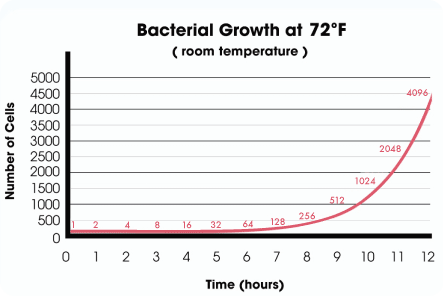# Bacteria: Math Lesson: Activity 1 of 3

## Directions

Bacteria multiply, or reproduce, by splitting in half. One cell grows and then divides into two cells. Two cells divide and become four cells. Four cells divide and become eight. Every time the bacteria divide, the total population doubles.

This is a line graph that shows how one lonely bacterial cell can multiply and become a colony of thousands within a day.Take a look at some important features of the graph.

• Title: tells what the graph is about
• Axis label: tells what the axis is measuring, with units
• x-axis: the horizontal axis (—), tells what is changing
• y-axis: the vertical axis (|), tells what we’re observing
• Scale: the numbers along each axis, which always follow a pattern
• Plot: the image (graph) representing the data, in this case the red line showing the number of bacteria over time
Question 1 of 8